The OpenFOAM Foundation
dynamicTreeDataPoint Class Reference

Holds (reference to) pointField. Encapsulation of data needed for octree searches. Used for searching for nearest point. No bounding boxes around points. Only overlaps and calcNearest are implemented, rest makes little sense. More...

## Public Member Functions

ClassName ("dynamicTreeDataPoint")

dynamicTreeDataPoint (const DynamicList< point > &points)
Construct from List. Holds reference! More...

label size () const

const DynamicList< point > & shapePoints () const
Get representative point cloud for all shapes inside. More...

volumeType getVolumeType (const dynamicIndexedOctree< dynamicTreeDataPoint > &, const point &) const
Get type (inside,outside,mixed,unknown) of point w.r.t. surface. More...

bool overlaps (const label index, const treeBoundBox &sampleBb) const
Does (bb of) shape at index overlap bb. More...

bool overlaps (const label index, const point &centre, const scalar radiusSqr) const
Check if any point on shape is inside sphere. More...

void findNearest (const labelUList &indices, const point &sample, scalar &nearestDistSqr, label &nearestIndex, point &nearestPoint) const
Calculates nearest (to sample) point in shape. More...

void findNearest (const labelUList &indices, const linePointRef &ln, treeBoundBox &tightest, label &minIndex, point &linePoint, point &nearestPoint) const
Calculates nearest (to line) point in shape. More...

bool intersects (const label index, const point &start, const point &end, point &result) const
Calculate intersection of shape with ray. Sets result. More...

## Detailed Description

Holds (reference to) pointField. Encapsulation of data needed for octree searches. Used for searching for nearest point. No bounding boxes around points. Only overlaps and calcNearest are implemented, rest makes little sense.

Optionally works on subset of points.

Source files

Definition at line 59 of file dynamicTreeDataPoint.H.

## Constructor & Destructor Documentation

 dynamicTreeDataPoint ( const DynamicList< point > & points )

Construct from List. Holds reference!

Definition at line 41 of file dynamicTreeDataPoint.C.

## Member Function Documentation

 ClassName ( "dynamicTreeDataPoint" )
 label size ( ) const
inline

Definition at line 81 of file dynamicTreeDataPoint.H.

Here is the call graph for this function: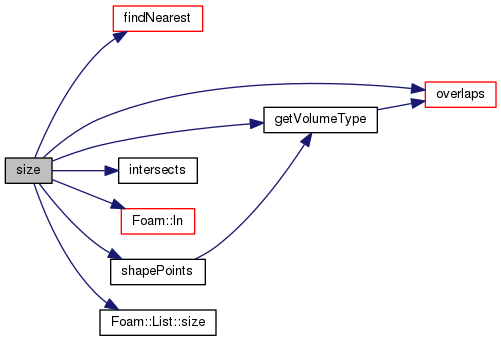const Foam::DynamicList< Foam::point > & shapePoints ( ) const

Get representative point cloud for all shapes inside.

(one point per shape)

Definition at line 52 of file dynamicTreeDataPoint.C.

References dynamicTreeDataPoint::getVolumeType().

Referenced by dynamicTreeDataPoint::size().

Here is the call graph for this function: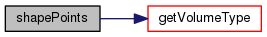Here is the caller graph for this function: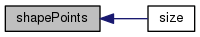Foam::volumeType getVolumeType ( const dynamicIndexedOctree< dynamicTreeDataPoint > & oc, const point & sample ) const

Get type (inside,outside,mixed,unknown) of point w.r.t. surface.

Only makes sense for closed surfaces.

Definition at line 59 of file dynamicTreeDataPoint.C.

References dynamicTreeDataPoint::overlaps(), and volumeType::UNKNOWN.

Referenced by dynamicTreeDataPoint::shapePoints(), and dynamicTreeDataPoint::size().

Here is the call graph for this function: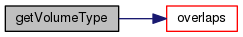Here is the caller graph for this function: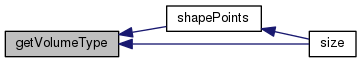bool overlaps ( const label index, const treeBoundBox & sampleBb ) const

Does (bb of) shape at index overlap bb.

Definition at line 69 of file dynamicTreeDataPoint.C.

References treeBoundBox::contains().

Referenced by dynamicTreeDataPoint::getVolumeType(), and dynamicTreeDataPoint::size().

Here is the call graph for this function: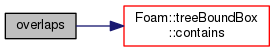Here is the caller graph for this function: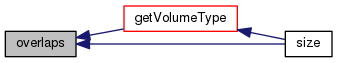bool overlaps ( const label index, const point & centre, const scalar radiusSqr ) const

Check if any point on shape is inside sphere.

Definition at line 79 of file dynamicTreeDataPoint.C.

References dynamicTreeDataPoint::findNearest(), Foam::magSqr(), and p.

Here is the call graph for this function: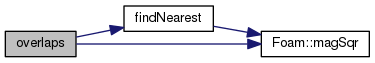void findNearest ( const labelUList & indices, const point & sample, scalar & nearestDistSqr, label & nearestIndex, point & nearestPoint ) const

Calculates nearest (to sample) point in shape.

Returns actual point and distance (squared)

Definition at line 99 of file dynamicTreeDataPoint.C.

References forAll, and Foam::magSqr().

Referenced by dynamicTreeDataPoint::overlaps(), and dynamicTreeDataPoint::size().

Here is the call graph for this function: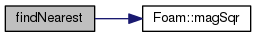Here is the caller graph for this function: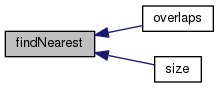void findNearest ( const labelUList & indices, const linePointRef & ln, treeBoundBox & tightest, label & minIndex, point & linePoint, point & nearestPoint ) const

Calculates nearest (to line) point in shape.

Returns point and distance (squared)

Definition at line 127 of file dynamicTreeDataPoint.C.

Here is the call graph for this function: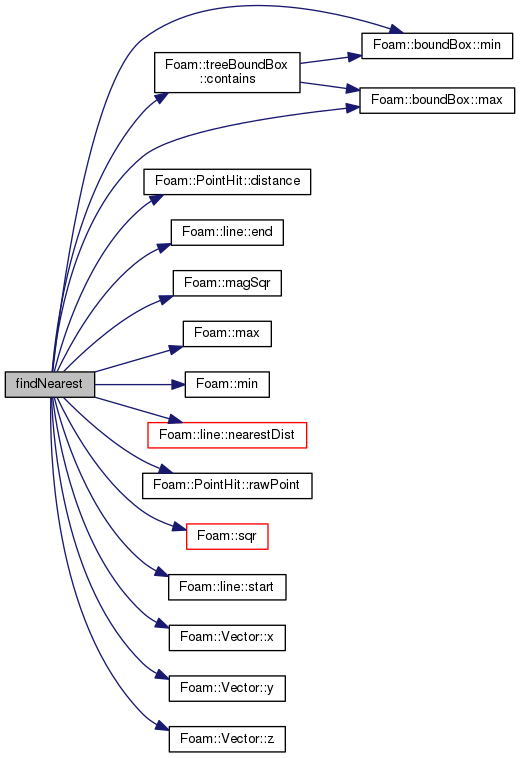bool intersects ( const label index, const point & start, const point & end, point & result ) const
inline

Calculate intersection of shape with ray. Sets result.

accordingly

Definition at line 144 of file dynamicTreeDataPoint.H.

References NotImplemented.

Referenced by dynamicTreeDataPoint::size().

Here is the caller graph for this function: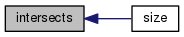The documentation for this class was generated from the following files: